Continuous-Time Aliasing Theorem Next  |  Prev  |  Up  |  Top  |  Index  |  JOS Index  |  JOS Pubs  |  JOS Home  |  Search

### Continuous-Time Aliasing Theorem

Let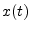denote any continuous-time signal having a Fourier Transform (FT)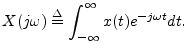Let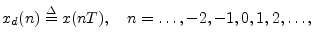denote the samples ofat uniform intervals of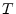seconds, and denote its Discrete-Time Fourier Transform (DTFT) by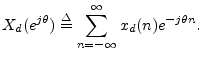Then the spectrum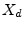of the sampled signal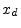is related to the spectrum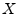of the original continuous-time signal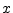by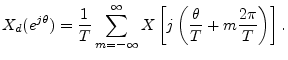The terms in the above sum for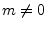are called aliasing terms. They are said to alias into the base band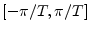. Note that the summation of a spectrum with aliasing components involves addition of complex numbers; therefore, aliasing components can be removed only if both their amplitude and phase are known.

Proof: Writingas an inverse FT gives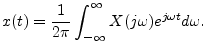Writing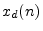as an inverse DTFT gives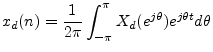where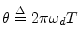denotes the normalized discrete-time frequency variable.

The inverse FT can be broken up into a sum of finite integrals, each of length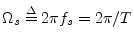, as follows: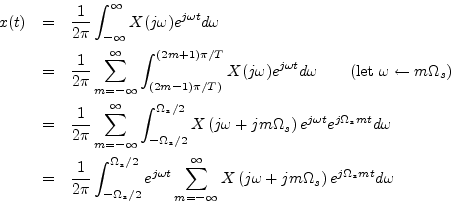Let us now sample this representation forat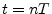to obtain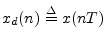, and we have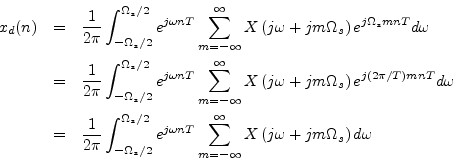since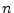and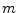are integers. Normalizing frequency as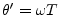yields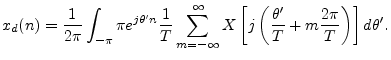Since this is formally the inverse DTFT of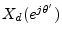written in terms of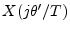, the result follows.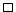Next  |  Prev  |  Up  |  Top  |  Index  |  JOS Index  |  JOS Pubs  |  JOS Home  |  Search

[How to cite this work] [Order a printed hardcopy]